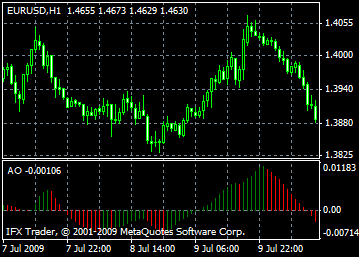# Awesome Oscillator - AO: description, adjustment and application

Indicator which is able to show what is happening with driving force of the market is technical indicator of Bill Williams Awesome Oscillator, AO. Indicator components: 34-period simple moving average constructed by the middle bar points (H+L)/2, which is subtracted from 5-period simple moving average, constructed by the middle bar points (H+L)/2.

#### Saucer

Being above zero mark, histogram gives the only signal for buying.

When histogram starts changing its direction from the lowest point to the highest one, when bar which is lower than the first one and red-colored, then "Saucer" signal appears.

For "Saucer" signal appearance it is necessary at least 3 bars of histogram.

Please note that using the "Saucer" signal for buying, Awesome Oscillator bars should be above zero line.

#### Zero crossing

When the histogram passes from negative rates to the positive ones, signal for buying appears.

Conditions:

only two bars are necessary for the signal;

one of the bars should be under zero level and the second one should cross it (pass from negative rate to the positive one).

There can be signal for buying and selling at the same time.

#### Two pikes

Being under the zero line the rate of histogram only in this case gives a signal for buying.

if the pike is downward (the lowest minimum), placed under the zero line and followed by another downward pike, which higher than the previous one (negative number which is less by absolute value, so it is close to zero line), it makes a signal.

Histogram must be placed between two pikes. Signal does not function in case histogram crosses zero line between the pikes. As soon as histogram crosses zero line, signal for buying appears immediately.

New pikes of histogram must be different by height, i.e. every new pike must be higher than previous one.

Additional signal for buying is formation of new higher pike; meanwhile histogram must not cross zero line.

#### Signals for selling

In Awesome Oscillator signals for selling are the same as signals for buying. Signal "Saucer" is inverted, placed lower than zero line. "Zero crossing" – first bar is higher than zero line, second bar is lower, i.e. descending. "Two pikes" is inverted and placed above zero line.#### Calculation

AO is a 34-period simple moving average, plotted through the central points of the bars (H+L)/2, and subtracted from the 5-period simple moving average, graphed across the central points of the bars (H+L)/2.

MEDIAN PRICE = (HIGH+LOW)/2

AO = SMA(MEDIAN PRICE, 5)-SMA(MEDIAN PRICE, 34)

Where:

SMA - Simple Moving Average.

Can't speak right now?